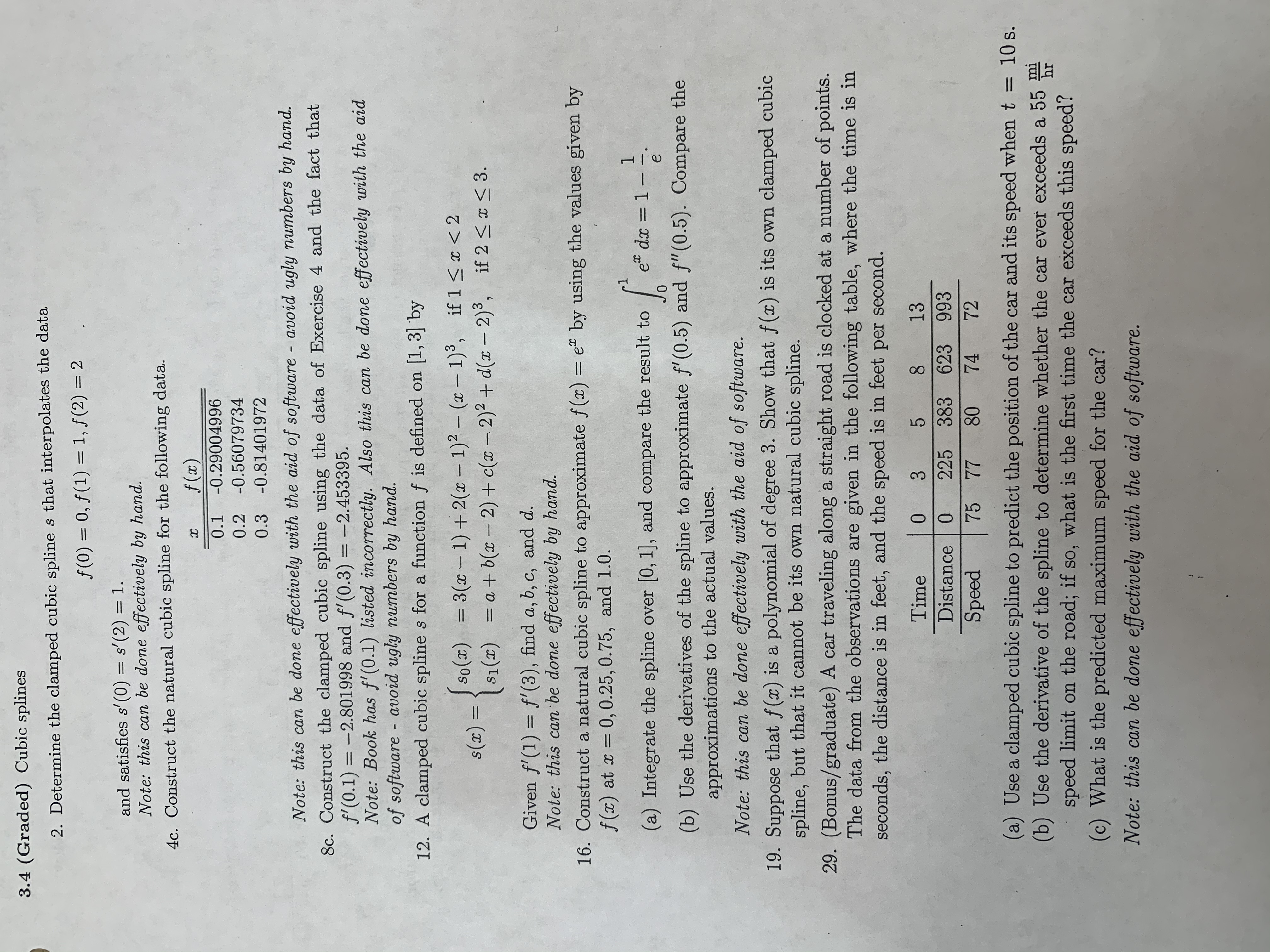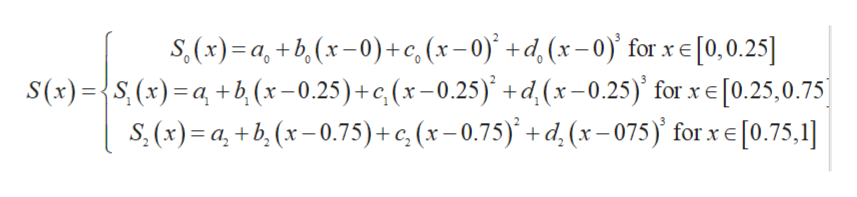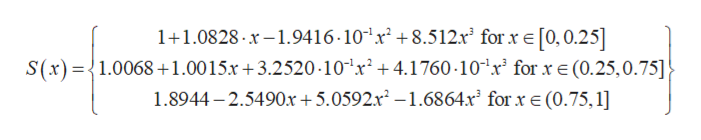# 3.4 (Graded) Cubic splines2. Determine the clamped cubic spline s that interpolates the dataf (0) 0, f(1) 1, f(2) 2and satisfies s' (0) = s'(2) = 1Note: this can be done effectively by hand.4c. Construct the natural cubic spline for the following data.f (x)х-0.290049960.1-0.560797340.2-0.814019720.3Note: this can be done effectively with the aid of software - avoid ugly numbers by hand.8c. Construct the clamped cubic spline using the data of Exercise 4 and the fact thatf'(0.1) =-2.801998 and f'(0.3) = -2.453395.Note: Book has f(0.1) listed incorrectly. Also this can be done effectively with the aidof software avoid ugly numbers by hand.12. A clamped cubic splines for a function f is defined on [1,3] byJso()3(-1)+2( -1)2 - (x - 1)3, if 1 < 2S1()a+b(x - 2)+ c( - 2)2 + d(x - 2)3,s(x) =if 2

Question
20 views

#16 from this handout

From Numerical Analysis 8th Edition by Richard Burden, Section 3.4help_outlineImage Transcriptionclose3.4 (Graded) Cubic splines 2. Determine the clamped cubic spline s that interpolates the data f (0) 0, f(1) 1, f(2) 2 and satisfies s' (0) = s'(2) = 1 Note: this can be done effectively by hand. 4c. Construct the natural cubic spline for the following data. f (x) х -0.29004996 0.1 -0.56079734 0.2 -0.81401972 0.3 Note: this can be done effectively with the aid of software - avoid ugly numbers by hand. 8c. Construct the clamped cubic spline using the data of Exercise 4 and the fact that f'(0.1) =-2.801998 and f'(0.3) = -2.453395. Note: Book has f(0.1) listed incorrectly. Also this can be done effectively with the aid of software avoid ugly numbers by hand. 12. A clamped cubic splines for a function f is defined on [1,3] by Jso()3(-1)+2( -1)2 - (x - 1)3, if 1 < 2 S1()a+b(x - 2)+ c( - 2)2 + d(x - 2)3, s(x) = if 2
check_circle

Step 1

Consider the function f(x) = ex and x0 = 0, x1 = 0.25, x2 = 0.75, x3 = 1.0

Let the polynomial passing through the above points:help_outlineImage Transcriptionclose.(x)a,+b(x-0) + c, (x - 0)' +d,(x-0) for x e [0,0.25] S(x)={S (x) ab, (x- 0.25) + c, (x - 0.25) +d(x-0.25) for x e[0.25,0.75 s. (x) a,+b (x-0.75) + c, (x - 0.75) + d (x-075) for x e [0.75, 1] fullscreen
Step 2

Then

a0 = f(x0) = f (0) = e0 = 1

a1 = f(x1) = f (0.25) = e.25 = 1.2840

a2 = f(x2) = f (0.75) = e0.75 = 2.1170

a3 = f(x3) = f (1) = e 1 = 2.7182

we have n = 3

h0 = x1 – x0 = 0.25

h1 = x2 – x1 = 0.50

h2 = x3 – x2 = 0.25

Step 3

Using the above conditions and ...help_outlineImage Transcriptionclose1+1.0828 x-.9416 10 x2 +8.5 12.x2 for xe[0,0.25] S(x)1.00681.0015.r 3.2520 10 x2 4.1760-10 r for xE (0.25,0.75] 1.8944-2.5490x +5.0592.x2-1.6864x2 for x e (0.75, 1] fullscreen

### Want to see the full answer?

See Solution

#### Want to see this answer and more?

Solutions are written by subject experts who are available 24/7. Questions are typically answered within 1 hour.*

See Solution
*Response times may vary by subject and question.
Tagged in

### Math